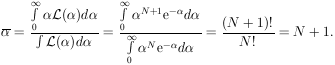### 13. POISSON DISTRIBUTION

A common type of problem which falls into this category is the determination of a cross section or a mean free path. For a mean free path, the probability of getting an event in an interval dx is dx /. Let P(0, x) be the probability of getting no events in a length x. Then we have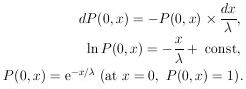(19)

Let P(N, x) be the probability of finding N events in a length x. An element of this probability is the joint probability of N events at dx1, ..., dxN times the probability of no events in the remaining length: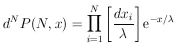(20)

The entire probability is obtained by integrating over the N-dimensional space. Note that the integral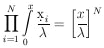does the job except that the particular probability element in Eq. (20) is swept through N! times. Dividing by N! gives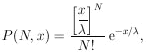the Poisson distribution (21)

As a check, note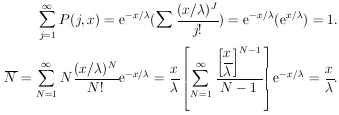Likewise it can be shown that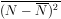=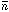. Equation (21) is often expressed in terms of: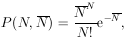the Poisson distribution (22)

This form is useful in analyzing counting experiments. Then the "true" counting rate is.

We now consider the case in which, in a certain experiment, N events were observed. The problem is to determine the maximum-likelihood solution forand its error:Thus we have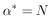and by Eq. (7),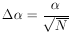In a cross-section determination, we have=x, whereis the number of target nuclei per cm3 and x is the total path length. Then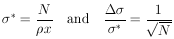In conclusion we note that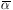: